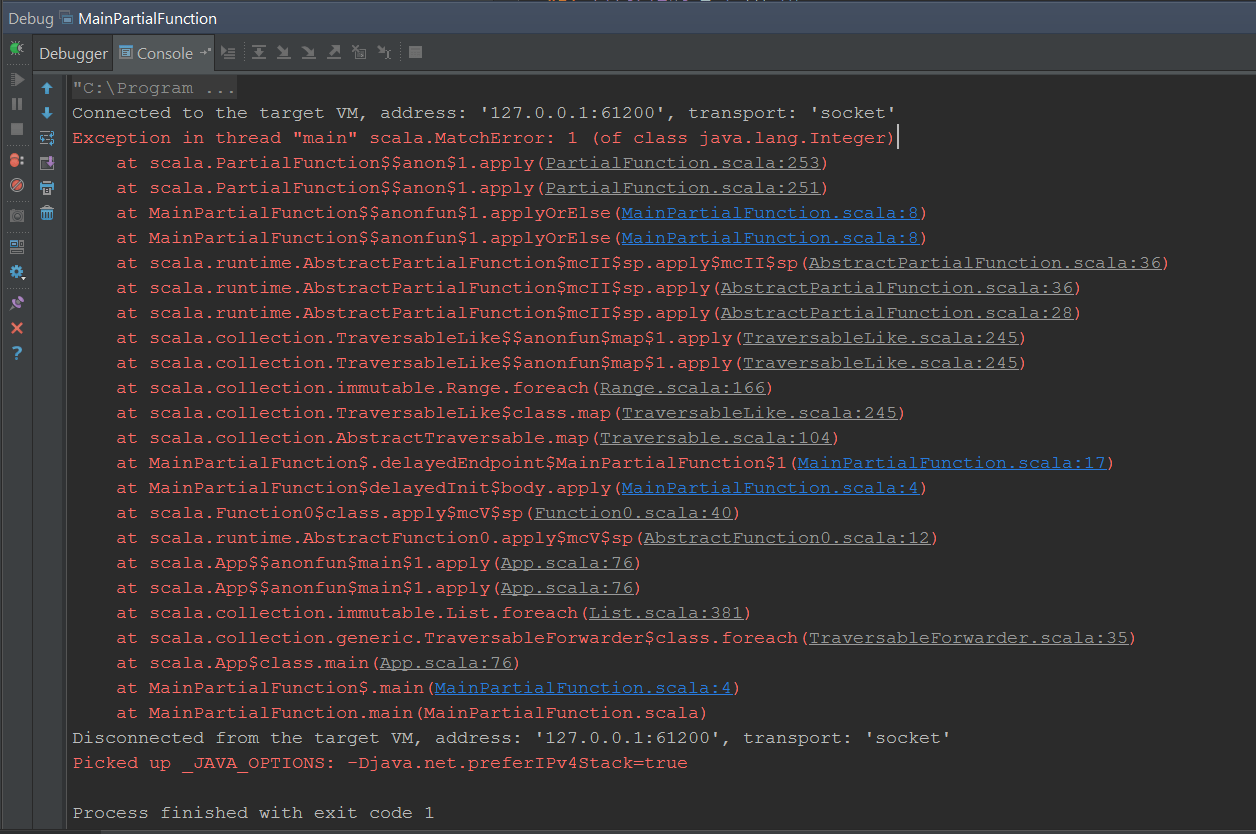By   June 22, 2015

PartialFunction (Partially defined functions) In Scala
A partial function is a unary function which is only defined for certain domain (i.e. input) values. They can be introduced anonymously. They can also be defined using PartialFunction trait.

In order to understand partial functions, first introduce a type Student. It has three properties, id, sType and name to keep information about identity, type and name of a student.

As discussed above, we can define partial functions anonymously or using PartialFunction trait. In the following example we have introduced two partial functions, fullTimeStudentNameFunction and partTimeStudentNameFunction, which would return the name of a full-time or part-time student respectively. Here we are also using the function to get the name of the student provided.

Debugging the above code provides the correct result and we get the name of students from their respective functions.

Since a partial function is defined for only certain input values. A non-supported input results in a run-time failure as follows:

Partial Functions usage in Scala’s collection API:
Scala’s collection API specially has a few methods which need partial functions for their operation. Just look at the intellisence in IntelliJ Idea. Here andThen and collect methods expect a partial function to work on items in the collection.

collect method filters and maps the element passing the filter. It is more concise than providing the definition of both filter and map functions.

Here is the output of the above code. It filters and maps correctly. We are using the result to print the names of the students.Let’s compare the results by providing a separate definition of filter and map. We can see that the results are the same in both cases.

## Using Partial Functions with Map

Although a partial function can be used with collection’s map function but if there is an input which is not supported by the partial function, it results in the same match-error.## Chaining Partial Functions

Partial functions can also be chained using andThen and OrElse. In the following example, we are chaining two partial functions to support the whole domain of student types.